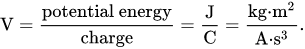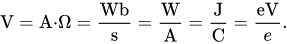# Volts to electron-volts free online calculator

Electrical voltage in volts (V) to energy in electron-volts (eV) calculator.

Enter the voltage in volts, charge in elementary charge or coulombs and press the Calculate button:

V
e
eV

eV to volts calculator »

## Volts to eV calculation with elementary charge

The energy E in electron-volts (eV) is equal to the voltage V in volts (V), times the electric charge Q in elementary charge or proton/electron charge (e):

E(eV) = V(V) × Q(e)

The elementary charge is the electric charge of 1 electron with the e symbol.

## Volts to eV calculation with coulombs

The energy E in electron-volts (eV) is equal to the voltage V in volts (V), times the electrical charge Q in coulombs (C) divided by 1.602176565×10-19:

E(eV) = V(V) × Q(C) / 1.602176565×10-19

### What is electronvolt (eV)?

In physics, an electronvolt (symbol eV, also written electron-volt and electron volt) is the amount of kinetic energy gained by a single electron accelerating from rest through an electric potential difference of one volt in vacuum. When used as a unit of energy, the numerical value of 1 eV in joules (symbol J) is equivalent to the numerical value of the charge of an electron in coulombs (symbol C). Under the 2019 redefinition of the SI base units, this sets 1 eV equal to the exact value 1.602176634×10−19 J.

Historically, the electronvolt was devised as a standard unit of measure through its usefulness in electrostatic particle accelerator sciences, because a particle with electric charge q has an energy E = qV after passing through the potential V; if q is quoted in integer units of the elementary charge and the potential in volts, one gets an energy in eV.

It is a common unit of energy within physics, widely used in solid state, atomic, nuclear, and particle physics. It is commonly used with the metric prefixes milli-, kilo-, mega-, giga-, tera-, peta- or exa- (meV, keV, MeV, GeV, TeV, PeV and EeV respectively). In some older documents, and in the name Bevatron, the symbol BeV is used, which stands for billion (109) electronvolts; it is equivalent to the GeV.

### What is volt?

The volt (symbol: V) is the derived unit for electric potential, electric potential difference (voltage), and electromotive force. It is named after the Italian physicist Alessandro Volta (1745–1827).

One volt is defined as the electric potential between two points of a conducting wire when an electric current of one ampere dissipates one watt of power between those points. Equivalently, it is the potential difference between two points that will impart one joule of energy per coulomb of charge that passes through it. It can be expressed in terms of SI base units (m, kg, s, and A) as:It can also be expressed as amperes times ohms (current times resistance, Ohm's law), webers per second (magnetic flux per time), watts per ampere (power per unit current, definition of electric power), or joules per coulomb (energy per unit charge), which is also equivalent to electronvolts per elementary charge:eV to volts calculator »

Currently, we have around 1975 calculators, conversion tables and usefull online tools and software features for students, teaching and teachers, designers and simply for everyone.